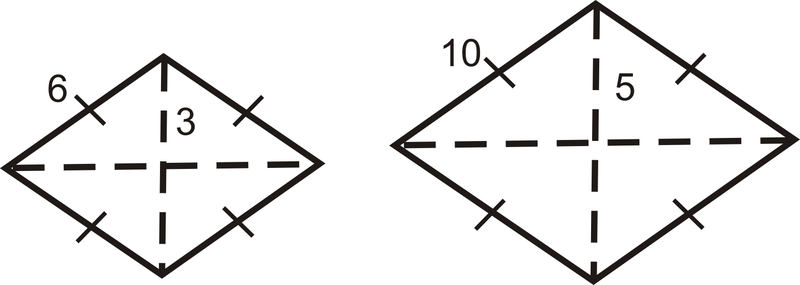# 5.22: Area and Perimeter of Similar Polygons

•• Contributed by CK12
• CK12

Ratio of the areas is the square of the scale factor; ratio of perimeters is the scale factor.

Polygons are similar when their corresponding angles are equal and their corresponding sides are in the same proportion. Just as their corresponding sides are in the same proportion, perimeters and areas of similar polygons have a special relationship.

Perimeters: The ratio of the perimeters is the same as the scale factor. In fact, the ratio of any part of two similar shapes (diagonals, medians, midsegments, altitudes, etc.) is the same as the scale factor.

Areas: If the scale factor of the sides of two similar polygons is $$\dfrac{m}{n}$$, then the ratio of the areas is $$\left(\dfrac{m}{n}\right)^2$$ (Area of Similar Polygons Theorem). You square the ratio because area is a two-dimensional measurement.Figure $$\PageIndex{1}$$

What if you were given two similar triangles and told what the scale factor of their sides was? How could you find the ratio of their perimeters and the ratio of their areas?

Example $$\PageIndex{1}$$

The two rectangles below are similar. Find the scale factor and the ratio of the perimeters and verify that the two results are the same.Figure $$\PageIndex{2}$$

Solution

The scale factor is $$\dfrac{16}{24}=\dfrac{2}{3}$$.

\begin{aligned}P_{\text{ small}}=2(10)+2(16)=52\text{ units }\\ P_{\text{ large}}=2(15)+2(24)=78\text{ units }\end{aligned}

The ratio of the perimeters is $$\dfrac{52}{78}=\dfrac{2}{3}$$.

Example $$\PageIndex{2}$$

Find the area of each rectangle from Example 1. Then, find the ratio of the areas and verify that it fits the Area of Similar Polygons Theorem.

Solution

\begin{aligned} A_{\text{ small}}&=10\cdot 16=160\text{ units}^2 \\ A_{\text{ large}}&=15\cdot 24=360\text{ units}^2 \end{aligned}

The ratio of the areas would be $$\dfrac{160}{360}=\dfrac{4}{9}$$.

The ratio of the sides, or scale factor is $$\dfrac{2}{3}$$ and the ratio of the areas is $$\dfrac{4}{9}$$. Notice that the ratio of the areas is the square of the scale factor.

Example $$\PageIndex{3}$$

Find the ratio of the areas of the rhombi below. The rhombi are similar.Figure $$\PageIndex{3}$$

Solution

Find the ratio of the sides and square it.

$$\left(\dfrac{3}{5}\right)^2=\dfrac{9}{25}$$

Example $$\PageIndex{4}$$

Two trapezoids are similar. If the scale factor is 34 and the area of the smaller trapezoid is $$81\text{ cm }^2$$, what is the area of the larger trapezoid?

Solution

First, the ratio of the areas is $$\left(\dfrac{3}{4}\right)^2=\dfrac{9}{16}$$. Now, we need the area of the larger trapezoid. To find this, set up a proportion using the area ratio.

\begin{aligned}\dfrac{9}{16}=\dfrac{81}{A} \rightarrow 9A&=1296 \\ A&=144\text{ cm }^2 \end{aligned}

Example $$\PageIndex{5}$$

Two triangles are similar. The ratio of the areas is $$\dfrac{25}{64}$$. What is the scale factor?

Solution

The scale factor is $$\sqrt{\dfrac{25}{64}}=\dfrac{5}{8}$$.

Example $$\PageIndex{6}$$

Using the ratios from Example 5, find the length of the base of the smaller triangle if the length of the base of the larger triangle is 24 units.

Solution

Set up a proportion using the scale factor.

\begin{aligned}\dfrac{5}{8}=\dfrac{b}{24} \rightarrow 8b&=120 \\ b&=15\text{ units } \end{aligned}

## Review

Determine the ratio of the areas, given the ratio of the sides of a polygon.

1. $$\dfrac{3}{5}$$
2. $$\dfrac{1}{4}$$
3. $$\dfrac{7}{2}$$
4. $$\dfrac{6}{11}$$

Determine the ratio of the sides of a polygon, given the ratio of the areas.

1. $$\dfrac{1}{36}$$
2. $$\dfrac{4}{81}$$
3. $$\dfrac{49}{9}$$
4. $$\dfrac{25}{144}$$

This is an equilateral triangle made up of 4 congruent equilateral triangles.

1. What is the ratio of the areas of the large triangle to one of the small triangles?Figure $$\PageIndex{4}$$
2. What is the scale factor of large to small triangle?
3. If the area of the large triangle is $$20\text{ units }^2$$, what is the area of a small triangle?
4. If the length of the altitude of a small triangle is $$2\sqrt{3}$$, find the perimeter of the large triangle.Figure $$\PageIndex{5}$$
5. Find the perimeter of the large square and the blue square.
6. Find the scale factor of the blue square and large square.
7. Find the ratio of their perimeters.
8. Find the area of the blue and large squares.
9. Find the ratio of their areas.
10. Find the length of the diagonals of the blue and large squares. Put them into a ratio. Which ratio is this the same as?
11. Two rectangles are similar with a scale factor of $$\dfrac{4}{7}$$. If the area of the larger rectangle is $$294\text{ in }^2$$, find the area of the smaller rectangle.
12. Two triangles are similar with a scale factor of $$\dfrac{1}{3}$$. If the area of the smaller triangle is $$22\text{ ft }^2$$, find the area of the larger triangle.
13. The ratio of the areas of two similar squares is $$\dfrac{16}{81}$$. If the length of a side of the smaller square is 24 units, find the length of a side in the larger square.
14. The ratio of the areas of two right triangles is $$\dfrac{4}{9}$$. If the length of the hypotenuse of the larger triangle is 48 units, find the length of the smaller triangle’s hypotenuse.

Questions 23-26 build off of each other. You may assume the problems are connected.

1. Two similar rhombi have areas of $$72\text{ units }^2$$ and $$162\text{ units }^2$$. Find the ratio of the areas.
2. Find the scale factor.
3. The diagonals in these rhombi are congruent. Find the length of the diagonals and the sides.
4. What type of rhombi are these quadrilaterals?

## Vocabulary

Term Definition
area The amount of space inside a figure. Area is measured in square units.
Perimeter Perimeter is the distance around a two-dimensional figure.
Proportion A proportion is an equation that shows two equivalent ratios.
Ratio A ratio is a comparison of two quantities that can be written in fraction form, with a colon or with the word “to”.
Scale Factor A scale factor is a ratio of the scale to the original or actual dimension written in simplest form.
Trapezoid A trapezoid is a quadrilateral with exactly one pair of parallel opposite sides.